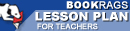Lesson Plans

# Journey Through Genius: The Great Theorems of Mathematics Quiz | Eight Week Quiz G

This set of Lesson Plans consists of approximately 142 pages of tests, essay questions, lessons, and other teaching materials.
 View a FREE sampleName: _________________________ Period: ___________________

This quiz consists of 5 multiple choice and 5 short answer questions through Cantor and the Transfinite Realm.

## Multiple Choice Questions

1. What was most useful about finding the square of a shape, before Hippocrates?
(a) It was useful in creating simple elevation maps,
(b) It was useful in finding the area of oddly shaped pieces of land.
(c) It was useful in determining the distance between two points.
(d) It was useful in finding the area of circles.

2. What was Eratosthanes most famous for?
(a) He showed that the Earth must be a sphere.
(b) He developed a simple way to find prime numbers and for determining the circumference of the Earth.
(c) He developed a way to navigate using logitude and latitude.
(d) He showed that there are no even prime numbers.

3. Which of the following is true about pi, as described by Dunham.
(a) The measurement of pi is no longer a mystery as we have an exact number value in modern mathematics.
(b) The measurement of pi should not have been so difficult for Archimedes to demonstrate.
(c) The measurement of pi was redetermined after Archimedes's death.
(d) The measurement of pi is a challenge that continues into modern mathematics.

4. What did Euclid state about pi in Elements?
(a) There is no relationship between the area of a circle and its circumference.
(b) There is a constant relationship between the area of a circle and the square of its diameter.
(c) The proportion of diameter to area is never equal.
(d) The proportion of area to circumference is never equal.

5. What were the main technique(s) that Euler used to find the sum of the series?
(a) Calculus methods.
(b) Cubic equations.
(c) Trigonometry and basic algebra.
(d) Quadratic sums,

## Short Answer Questions

1. What was most noticeable about Euler at a young age?

2. In what time period did mathematicians find a solution to cubic equations?

3. What did Dunham describe about the following series 1 + 2 + 3 + 4. . .?

4. What did most of 19th century mathematics focus on, as highlighted by Dunham?

5. Who was Heron?

(see the answer key)

 This section contains 375 words (approx. 2 pages at 300 words per page) View a FREE sampleCopyrightsJourney Through Genius: The Great Theorems of Mathematics from BookRags. (c)2019 BookRags, Inc. All rights reserved.
Follow Us on Facebook